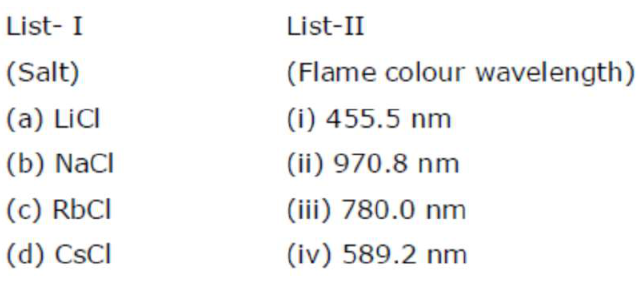# Match List-I with List-II

Question:

Match List-I with List-IIChoose the correct answer from the options given below :

1. $(\mathrm{a})-(\mathrm{ii}),(\mathrm{b})-(\mathrm{i}),(\mathrm{c})-(\mathrm{i} v),(\mathrm{d})-(\mathrm{iii})$

2. (a) $-($ ii $),(b)-($ iv $),(c)-($ iii $),(d)-($ i $)$

3. (a) $-($ iv $),($ b $)-($ ii $),($ c $)-($ iii $),($ d $)-($ i $)$

4. $(\mathrm{a})-(\mathrm{i}),(\mathrm{b})-(\mathrm{i} v),(\mathrm{c})-(\mathrm{ii}),(\mathrm{d})-(\mathrm{iii})$

Correct Option: , 2

Solution:

Range of visible region :

$390 n m-760 n m$

VIBGYOR

Violet Red

LiCl Crimson Red

$\mathrm{NaCl}$ Golden yellow

$\mathrm{RbCl}$ Violet

CsCl Blue

So Lid Which is crimson have wave length closed to red in the spectrum of visible region which is as per given data is.